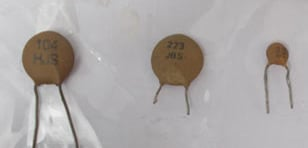# Capacitor Value Calculator

This Capacitor Value Calculator calculates the capacitance value of a ceramic capacitor upon providing the capacitor code in the input field below.

## Capacitor Code Calculator

This Capacitor Code Calculator calculates the ceramic capacitor code upon providing the capacitance value of the capacitor in the input field below.

### How Capacitor Value/Code Calculator Works?

As ceramic capacitors have less surface area because of their tiny size, their value is not written in the capacitor, instead an encoded code is written on them. By using this capacitor value calculator, we can calculate the value of that capacitor, or vice versa. For electrolytic capacitors, simply capacitance values are written on them.

Encoding for Ceramic Capacitors

Ceramic capacitors encoding consists 1-3 digits.

If the capacitor code consist only 1 or 2 digits, it is simply their capacitance value in PicoFarads (pF). For example if a ceramic capacitor has a code ‘5’ and other has ‘47’, their respective capacitance values are 5 pF and 47 pF.For 3 digit’s capacitor code, the first two digits are the capacitance value in pF and the third digit is a multiplier factor of first two digits to calculate the final capacitance value of the capacitor.

The 3rd digit ranges between 0-6. It can’t exceed more than 6.

If 3rd digit is 0, it means multiplier factor of 1.

If 3rd digit is 1, it means multiplier factor of 10.

If 3rd digit is 2, it means multiplier factor of 100.

If 3rd digit is 3, it means multiplier factor of 1000.

If 3rd digit is 4, it means multiplier factor of 10000.

If 3rd digit is 5, it means multiplier factor of 100000.

If 3rd digit is 6, it means multiplier factor of 1000000.

To understand how multiplier works, let’s take an example of a capacitor with code ‘104’.

As first two digits are 10 and the 3rd digit is 4, so multiplier factor is 10000, the total capacitance value in pF is as follows:

10*10000 = 100000 pF

Similarly if the capacitor code is 152, the 3rd digit is 2 so multiplier factor is 100. The capacitance value will be calculated as follows:

15*100 = 1500 pF

So this is how a capacitor value/code calculator calculates the ceramic capacitor value from the capacitor code, or vice versa.